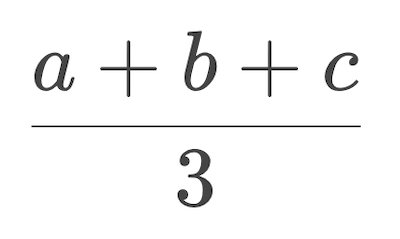# Return

New:

• `return` statement

To fix our problem and make the function work properly we need a new keyword: `return`. Put it in front of the calculation expression, keeping the indentation of this line to 4 spaces:

``````def get_power(base, power):
return base ** power

print(get_power(3, 2))
``````
```9
```

Success! At least in this simple scenario our brand new function works like `pow`. That's the generating of a value we've talked about in the previous course. The proper term is "returning a value". The word "return" is used in programming for historical reasons. It means to generate a value and let the interpreter return from the body to the position where the call was written, and to continue executing code from there (this will be discussed in "Flow of execution"). From now on, we'll refer to values generated by functions as "returned values".

Let's also do what we did in the previous course and save the result of calling a function into a variable:

``````def get_power(base, power):
return base ** power

x = get_power(3, 2)
print(x)
``````
```9
```

Now you can use the variable `x` instead of the full function call later in the program. Great! You have successfully created your own, fully working Python function. Now let's build a few other functions that return useful answers.

### Example 1: average calculator

An average of three numbers can be calculated using the formula:A Python function which reflects this formula looks like so:

``````def get_average(a, b, c):
return (a + b + c) / 3

print(get_average(4, 8, 27))
``````
```21
```

The number `3` is fixed in the formula, so we fix it in the function's body rather than pass via a parameter. It is always `3`, and the whole purpose of parameters is to allow changeable values.

(Note the use of parentheses in the calculation. They are needed for priority, just like in arithmetic, because we need to sum the numbers first, and then divide the sum by 3.)

### Example 2: strings gluer

This function takes two strings and glues them together: the second string in front, the first string in the end.

``````def glue_strings(a, b):
return b + a

print(glue_strings("See", "Bee"))
``````
```BeeSee
```

This function is meant to work with strings only. Since the concatenation (gluing) is the same symbol `+` as mathematical addition, `glue_strings` can accept numbers as arguments, and return their sum. However, doing so would be considered a logical mistake. Technically works, but conceptually doesn't make sense because the name so clearly points to gluing strings and nothing else.

### Example 3: get_power in two lines

So far, the bodies of our functions each contained only one line of code. Functions are sort of sub-programs, they may contain any amount of code needed. Any expression and almost any statement can be a part of a function's body. Consider this alternative, two-lines implementation of the same `get_power`:

``````def get_power(base, power):
result = base ** power
return result

print(get_power(3, 2))
``````

This example also demonstrates a simple case where the returning value is derived from a variable (rather than a complex expression like a math operation). A single variable is one of the simplest expressions possible.